Next: Distance Problems Up: Applications/New Directions Previous: Clique Graphsk-null Ordered

## Order vs. Analysis

The cornerstone of applications of order-theoretical fixed point methods to analysis so far appears to be the Abian-Brown-Pelczar theorem:   In a chain-complete ordered set P every order-preserving map for which there is a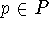withhas a fixed point. Abian and Brown prove it in  as a result on ordered sets. Pelczar in the introduction of  mentions the possible application to integral inequalities, but gives no specific examples. Recently these methods have been used sucessfully by Heikkilä, Lakhshmikantham, Carl and Sun to prove existence results for solutions for various differential and integral equations (cf. , , -). Similar iterations are used by Heikkilä and Salonen to derive results in game theory (cf. ). Due to the often very specific nature of results in nonlinear analysis we will only present one example and the order-theoretical iteration method that is used. The presentation is only intended to give a general idea of the arguments (the order-theoretical results can be derived fairly quickly and for a complete introduction to nonlinear analysis there certainly is not enough room in this paper). For precise proofs the reader is referred to . For a larger set of results and references cf. the text .
The underlying order-theoretical iteration used is similar in spirit to Abian's f-chains (cf. ) respectively Cousot and Cousot's iteration in  and . Note however that it is neither assumed here that the function is order-preserving, nor that the underlying set is chain-complete.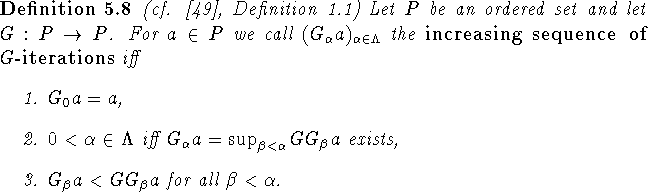Clearly this sequence would be the maximal sequence generated by a transfinite iteration scheme (cf. , Lemma 1.1) such that

1.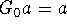,
2.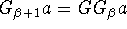iff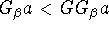,
3.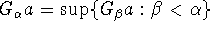if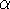is a limit ordinal,
where the iteration stops when one of the steps 2 or 3 cannot be carried out.As noted in Theorem 1.1 in , the increasing sequence of G-iterations always exists and is unique. Moreover the proof of this result does not depend on the Axiom of Choice (a similar point was made in  for the Abian-Brown-Pelczar theorem). Unfortunately due to the extremely weak hypotheses the sequence might have only one element, which need not be a fixed point. However (, Lemma 2.1) if the increasing sequence of G-iterations has a last element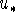such that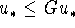, thenis a fixed point of G. (Otherwise we could extend the sequence by addingcontradicting the maximality of the sequence.)
Unfortunately this result still is too general to be of much apparent use, as the iteration could stop at any successor or limit ordinal and the stopping need not guarantee a fixed point. Introducing the hypothesis of being order-preserving however one obtains the following variant of the Abian-Brown-Pelczar theorem: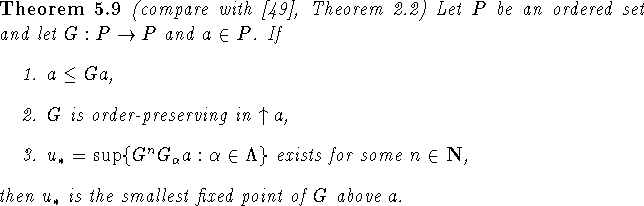Condition 2 insures that nothing goes wrong at successor ordinals and condition 3 insures the existence of all needed suprema. Condition 3 looks a little strange to the order-theorist, especially considering that (, Lemma 2.2) it is equivalent to the simpler condition 3 with n=0. However when applying the result in analysis it is sometimes easier to prove condition 3 for an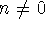than it would be to prove it for n=0. The included example of a Hammerstein integral equation is an example. This explains the format of condition 3.
It would be interesting to see how far this idea can be taken under mild hypotheses on the underlying ordered set. It is easy to see that every space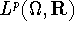, where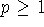and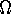is a measure space, is conditionally complete   (i.e., every set with an upper bound has a lowest upper bound) with the pointwise almost everywhere order. Thus conditional completeness might be a good hypothesis. Then one could try to devise an iteration as described above also for the case for which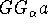is not comparable to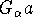: As long as all is bounded above, take the supremum as the next element of the sequence. This is similar to the approach in , yet the author would hope that milder conditions than assuming the underlying set is a complete lattice would lead to success.
As an example let us consider the following Hammerstein integral equation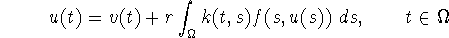which is considered in , section 3.is a closed and bounded subset of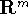and all functions except k assume values in an ordered Banach space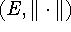with a closed and regular positive cone K. (One could envision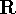with its natural order here to assimilate the general flavor of the following.) Now assume:

1.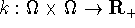(i.e., k does not take negative values) is continuous,
2.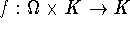(i.e., f takes values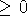in E) is such that
1.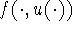is strongly measurable for each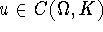,
2.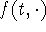is increasing for almost every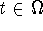,
3.   There are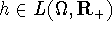and a Bochner-integrable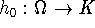such that for all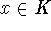and almost everywe have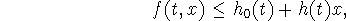3.   r>0 is such that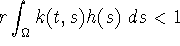for each.
Then for each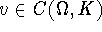the above integral equation has a solution.
Clearly the operator to work with is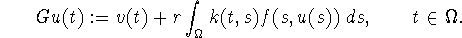The fact that k maps into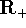and f maps into K insures that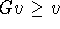. Condition 2b insures that G is order-preserving. Condition 3 together with condition 2c insures (via an analytical argument) that there is function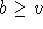such that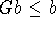. Thus G maps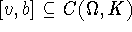to itself. Unfortunately this interval is not chain-complete, so we are not trivially done. As done in section 3 of  a short analytical argument shows that condition 3 of Theorem 5.9 is satisfied with n=1. This proves that G has a fixed point and thus that the equation in question has a solution.

It can be proved that the set of all functions on [0,1] that are constant on intervals bounded by dyadic rationals and take dyadic rational numbers as values forms a pseudo cone as defined in . This construction is such that the restriction of the pseudo cone structure to natural subsets, such as functions constant on intervals bounded by dyadic rationals of order n (denominator at most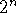), taking dyadic rational numbers of order n as values, also is a pseudo cone. The domain [0,1] is chosen for convenience only. Thus there is a possibility that Baclawski's algorithm for pseudo cones can have further impact on the use of order in analysis.Next: Distance Problems Up: Applications/New Directions Previous: Clique Graphsk-null Ordered

Bernd.S.W.Schroder